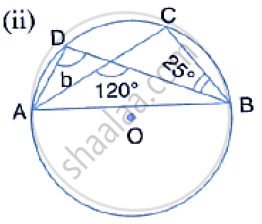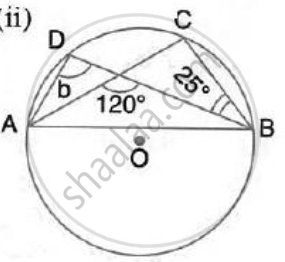Share

In the Following Figures, O is the Centre of the Circle. Find the Values of A, B, C and D. - ICSE Class 10 - Mathematics

ConceptChord Properties - Chords Equidistant from the Center Are Equal (Without Proof)

Question

In the following figures, O is the centre of the circle. Find the values of a, b, c and d.SolutionHere, ∠DAC = ∠CBD = 25°
(Angle subtended by the same chord on the circle are equal)

Again, 120°= b + 25°
(In a triangle, measure of exterior angle is equal to the sum of pair of opposite interior angles)
⇒ b = 95°

Is there an error in this question or solution?

Video TutorialsVIEW ALL 

Solution In the Following Figures, O is the Centre of the Circle. Find the Values of A, B, C and D. Concept: Chord Properties - Chords Equidistant from the Center Are Equal (Without Proof).
S